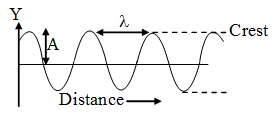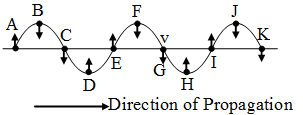Ex﻿

# 5. Sound

#### Terms Used For Defining Wave Motion

Wave Length : The distance between two nearest points in a wave which are in the same phase of vibration is called the wave length. In simple words it is the length of one complete wave. It is denoted by λ.Amplitude :The amplitude of a wave is the magnitude of maximum displacement of the vibrating particles on the either side of their mean position. It is denoted by the letter A and its SI unit is metre (m).
Time-Period : The time required to produce one complete wave (or cycle) is called time-period of the wave.
Frequency : The frequency of an oscillating particle is the number of oscillations completed in one second. The unit of frequency is hertz (or Hz). The frequency of the wave is 1\T. It is generally represented by ν (nu).
Wave Velocity : The distance travelled by the wave in one second is called the wave velocity. It is represented by 'v' and its unit is ms–1.
Phase :All the points on a wave which are in the same state of vibration are said to be in the same phase. Thus, in the wave shown in fig.(a) Points B, F and J are in the same phase – all lie on the crests.
(b) Points D and H are in the same phase – both lie on the troughs.
(c) Points A, E and I are in the same phase. All these points are just about to start their vibration in the upward direction from their mean positions.
(d) Points C, G and K are in the same phase. All these points are just about to start their vibration in the downward direction from their mean positions.

If you want to give information about online courses to other students, then share it with more and more on Facebook, Twitter, Google Plus. The more the shares will be, the more students will benefit. The share buttons are given below for your convenience.
×

#### NTSE Physics (Class X)

• Electricity
• Magnetic Effect of Curent
• Light Reflection
• Light Refraction
• Human Eye & Colourful World
• Source of Energy
• Motion

#### NTSE Physics (Class IX)

• Force & laws of motion
• Work and Energy
• Gravitation
• Sound

SHOW CHAPTERS